# Number

Determine unknown number if you know that difference between five times and triple of number is 42.

x =  21

### Step-by-step explanation:

5x-3x = 42

5•x-3•x = 42

2x = 42

x = 21

Our simple equation calculator calculates it.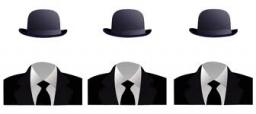We will be pleased if You send us any improvements to this math problem. Thank you!Tips to related online calculators
Do you have a linear equation or system of equations and looking for its solution? Or do you have a quadratic equation?

## Related math problems and questions:

• One-thirdA one-third of unknown number is equal to five times as great as the difference of the same unknown number and number 28. Determine the unknown number.
• Unknown number 24I think the number: a - is the same as the square area that has the 12th circumference. What is this number? b - its half is 7 times bigger than its quarter. Is this the number?
• Unknown numberFind an unknown number of which 1/5 is 40 greater than one-tenth of that number.
• Birds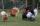On the farm they have a total of 110 birds. Geese and turkeys together is 47. Hens is three times more than the turkey. How much is poultry by species?
• Unknown numberUnknown number is divisible by exactly three different primes. When we compare these primes in ascending order, the following applies: • Difference first and second prime number is half the difference between the third and second prime numbers. • The prod
• Unknown number xyzFind the number that its triple is 24. Solve by equation.
• Unknown number 16My number's tens is three times more then ones My number's ones is twice the number of thousands and my number's hundreds is half the number of of tens. I have two ones. which number am I?
• Unknown number 2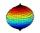I think the number. When he reduces it four times, I'll get 11. What number am I thinking?
• The number 3Ski organizers should print the start numbers from 1 to 45. How many times will they use the number 3 when printing?
• Number unknownAdela thought the two-digit number, she added it to its ten times and got 407. What number does she think?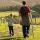Daddy is a 46 year old and son is at age of 16. When (which year) was/will daddy 5 times more years than his son?I think number.When I add 841 to it and subtract 157, I get a number that is 22 greater than 996. What number I thinking?I think the number: Its half is 16 bigger than his quarter. I think the number N . ..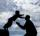Father is 44 years old, his son 16 years. Determine how many years ago was the father five times older than the son.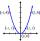Calculate unknown number whose 12th power when divided by the 9th power get a number 27 times greater than the unknown number. Determine the unknown number.A number decreased by the difference between four and the number.Common multiple of three numbers is 3276. One number is in this number 63 times, second 7 times, third 9 times. What are the numbers?